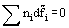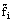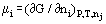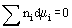# GIBBS-DUHEM EQUATION

There are several Gibbs-Duhem equations, although the name is most commonly associated with just one of them. They refer to the properties of fluid (gas or liquid) mixtures at fixed pressure and temperature. The equations all have the same form, namely:
(1)whereis a partial molar quantity for component i, ni is the number of moles of i present in the mixture and the summation is over all components present in the mixture,can be any partial molar quantity, volume, enthalpy, entropy etc., but the one of greatest utility is the partial molar Gibbs free energy, usually called the Chemical Potential, μ, defined by:
(2)where G is the Gibbs Free Energy and nj in the constraint list indicates that all amounts of substance are to be held fixed, except for ni, when performing the differentiation.
The Gibbs-Duhem equation for this partial molar quantity, and the one most normally associated with the name, is then:
(2)This equation shows that the chemical potentials in a mixture are not all independent and that there is a constraint equation which they must satisfy.